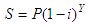# Salvage Value Calculator

At the bottom you can find a Salvage Value Calculator. The term means the estimated value that an asset will get upon its sale at the end of its useful life. This value is used in accounting to determine depreciation amounts and to determine deductions. The value can be a guess of the end value or can be determined by an official regulatory entity.

The value is obtained by the following formula:where:

S = salvage value
P = original price
i = nominal depreciation rate
Y = age in years

### Car Salvage Value - Example

What is the salvage value of Monique's high-end car if it's four years old, she bought it for \$49,500 and it depreciates 23% annually?

Let's use our calculator and enter...

Original Price = 49,500.00
Depreciation rate = 23%
Number of years =  4

the result is

Salvage Value =     \$17,400.76

 To be able to use the following calculator, you have to allow JavaScript codes on your navigator.

 Enter the needed values... (do not use commas) Original Price: Depreciation Rate: % Number of Years: Salvage Value:

From 'Salvage Value Calculator' to home

From 'Salvage Value Calculator' to Free Online Calculators